# Division + divisibility - math problems

#### Number of problems found: 71

• DivisibilityDetermine all divisors of number 84.
• DivisibilityWrite all the integers x divisible by seven and eight at the same time for which the following applies: 100 < x < 200.
• RemainderA is an arbitrary integer that gives remainder 1 in the division with 6. B is an arbitrary integer that gives remainder 2 the division by. What makes remainder in division by 3 product of numbers A x B ?
• CalculateCalculate the sum of all three-digit natural numbers divisible by five.
• Numbers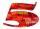Write smallest three-digit number, which in division 5 and 7 gives the rest 2.
• Divisors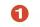Find all divisors of number 493. How many are them?
• No. of divisorsHow many different divisors have number ??
• Twenty-fiveHow many three-digit natural numbers are divisible by 25?
• Hens and pigs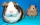Hens and pigs have 46 feet in total. At least how much can heads have?
• Lcm 2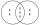Create the smallest possible number that is divisible by numbers 5,8,9,4,3
• Decomposition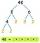Make decomposition using prime numbers of number 155. Result write as prime factors (all, even multiple)
• Count of roots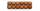How many solutions has equation x. y = 7757 with two unknowns on the set of natural numbers?
• Divisible by nineHow many three-digit natural numbers in total are divisible without a remainder by the number 9?
• DivisibilityIs the number 761082 exactly divisible by 9? (the result is the integer and/or remainder is zero)
• Troops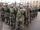If the general sorts troops into the crowd by nine left 6. How many soldiers has regiment if we know that they are less than 300?
• Prime divisorsFind 2/3 of the ratio of the sum and the product of all prime divisors of the number 120.
• Banknotes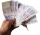How many different ways can the cashier payout € 310 if he uses only 50 and 20 euro banknotes? Find all solutions.
• PlumsIn the bowl are plums. How many would be there if we can divide it equally among 8, 10 and 11 children?
• Stacks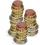Annie has a total of \$ 702. The money must be divided into stacks so that each buyer has the same amounth of dollars. How many options she have?
• 7 digit numberIf 3c54d10 is divisible by 330, what is the sum of c and d?

Do you have an interesting mathematical word problem that you can't solve it? Submit a math problem, and we can try to solve it.

We will send a solution to your e-mail address. Solved examples are also published here. Please enter the e-mail correctly and check whether you don't have a full mailbox.

Please do not submit problems from current active competitions such as Mathematical Olympiad, correspondence seminars etc...

Division Problems. Divisibility - math word problems.